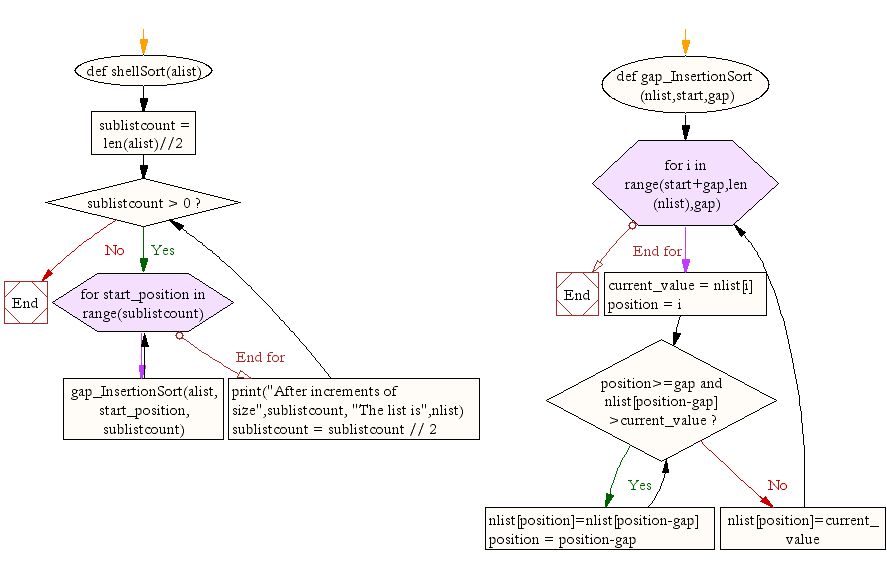﻿ Python Data Structures and Algorithms: Shell sort - w3resource# Python Data Structures and Algorithms: Shell sort

## Python Search and Sorting: Exercise-7 with Solution

Write a Python program to sort a list of elements using shell sort algorithm.
Note : According to Wikipedia "Shell sort or Shell's method, is an in-place comparison sort. It can be seen as either a generalization of sorting by exchange (bubble sort) or sorting by insertion (insertion sort). The method starts by sorting pairs of elements far apart from each other, then progressively reducing the gap between elements to be compared. Starting with far apart elements can move some out-of-place elements into position faster than a simple nearest neighbor exchange."

Sample Solution:

Python Code:

``````def shellSort(alist):
sublistcount = len(alist)//2
while sublistcount > 0:
for start_position in range(sublistcount):
gap_InsertionSort(alist, start_position, sublistcount)

print("After increments of size",sublistcount, "The list is",nlist)

sublistcount = sublistcount // 2

def gap_InsertionSort(nlist,start,gap):
for i in range(start+gap,len(nlist),gap):

current_value = nlist[i]
position = i

while position>=gap and nlist[position-gap]>current_value:
nlist[position]=nlist[position-gap]
position = position-gap

nlist[position]=current_value

nlist = [14,46,43,27,57,41,45,21,70]
shellSort(nlist)
print(nlist)
```
```

Sample Output:

```After increments of size 4 The list is [14, 41, 43, 21, 57, 46, 45, 27, 70]
After increments of size 2 The list is [14, 21, 43, 27, 45, 41, 57, 46, 70]
After increments of size 1 The list is [14, 21, 27, 41, 43, 45, 46, 57, 70]
[14, 21, 27, 41, 43, 45, 46, 57, 70]
```

Flowchart:## Visualize Python code execution:

The following tool visualize what the computer is doing step-by-step as it executes the said program:

Python Code Editor:

What is the difficulty level of this exercise?

Test your Python skills with w3resource's quiz

﻿

## Python: Tips of the Day

Returns the maximum value of a list, after mapping each element to a value using the provided function

Example:

```def tips_max(lst, fn):
return max(map(fn, lst))

print(tips_max([{ 'n': 4 }, { 'n': 2 }, { 'n': 8 }, { 'n': 6 }], lambda v : v['n']))
```

Output:

```8
```